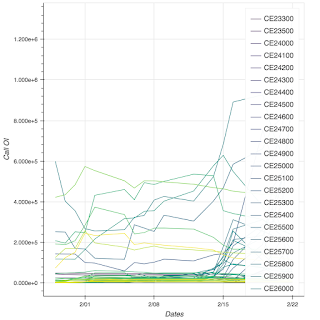# multi_line() legend with colors assigned using linear_cmap

Hi,

I’m using multi_line() to create a set of lines and the colors to those lines are assigned using a linear_cmap that transforms values from a ColumnDataSource field to colors for those lines. However, the legend created displays a single color against all the legend entries.

call_chain_plot=figure()

``

callSource = ColumnDataSource(data=dict(

``

``````xs=[bnf.TIMESTAMP.tolist() for i in range(0, len(call_strikes))],     # x coords for each line (list of lists)
``````

``

``````ys=[bnf[strike].tolist() for strike in call_strikes],                 # y coords for each line (list of lists)
``````

``

``````call_strike_vals=call_strike_vals,                                    # data to use for colormapping
``````

``

``````labels = call_strikes
``````

``

))

``

call_chain_plot = figure(y_axis_label=‘Call OI’, x_axis_label=‘Dates’, x_axis_type = ‘datetime’)

``

x=call_chain_plot.multi_line(‘xs’, ‘ys’, source=callSource, color=linear_cmap(‘call_strike_vals’, “Viridis256”, min(call_strike_vals), max(call_strike_vals)), legend=‘labels’)

``

The output isAlso, is there a way to reposition the legend to prevent it from overlapping with the chart area?